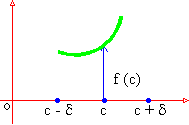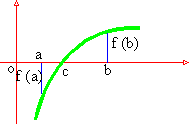Home MonkeyNotes Printable Notes Digital Library Study Guides Study Smart Parents Tips College Planning Test Prep Fun Zone Help / FAQ How to Cite New Title Request

 3.6 Properties Of Functions Continuous On An Interval Property 1: If a function f is continuous on [ a, b ], then the graph of the function is unbroken ( i.e. continuous) curve joining the points (a, f (a) ) and (b, f ( b))Property 2: If f is the continuous on [ a, b ] and f ( c ) ¹ 0 and a < c < b, then there exists a small positive number d. So that f ( x ) is of the same sign as f ( c ) then c - d < x < c + d. i.e. a continuous function does not undergo sudden changes. This property will be later on used for the discussion of Maxima and Minima.Property 3: If f is continuous on [a , b] and f (a), f (b) are opposite signs, then f (x) = 0 for at least one value of x in (a , b).   Property 4: If f is continuous on a closed interval [a , b] then i) f is bounded in [ a, b ] ii) There exists points c and d in [ a, b] where f assumes its lub, and glb, M and m respectively. i.e. f (c) = M and f (d) = m iii) f assumes every value between m and M at least one value x [ a, b ] Note : 1) A discontinuous function may be bounded but may not attain the glb or lub. Even a continuous function on an open interval may not attain glb and lub. 2) These properties are just the necessary conditions but not the sufficient condition to make a function continuous. ********** Index 3.1 Continuity At a Point 3.2 Continuity In An Interval 3.3 Some Very -often - encountered Continuous Functions 3.4 Algebra Of Continuous Functions 3.5 Discontinuity And its Classification 3.6 Properties of Functions Continuous on an Interval Chapter 4 Follow @Pinkmonkey_comYour browser does not support the IFRAME tag.